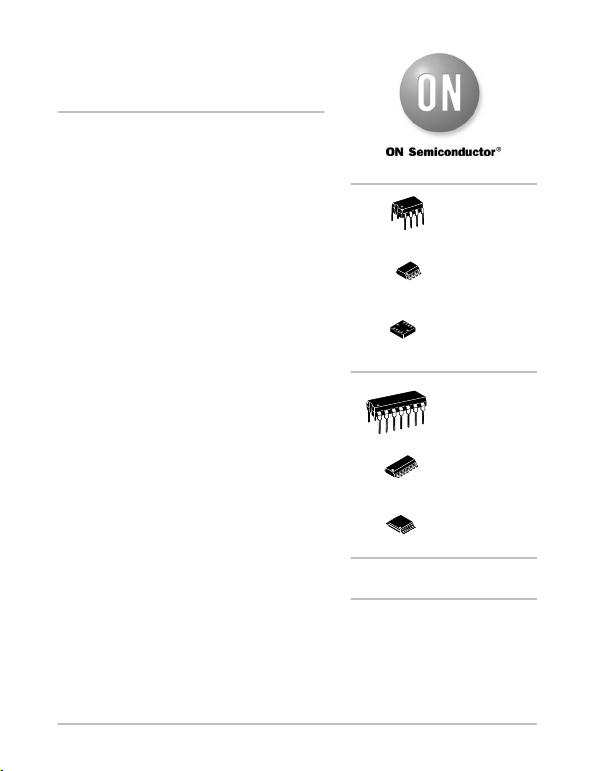© Semiconductor Components Industries, LLC, 2012
August, 2012 Rev. 18
1Publication Order Number:
MC34071/D
MC34071,2,4,A
MC33071,2,4,A,
NCV33072,4,A
Single Supply 3.0 V to 44 V
Operational Amplifiers
Quality bipolar fabrication with innovative design concepts are
employed for the MC33071/72/74, MC34071/72/74, NCV33072/74A
series of monolithic operational amplifiers. This series of operational
amplifiers offer 4.5 MHz of gain bandwidth product, 13 V/ms slew rate
and fast settling time without the use of JFET device technology.
Although this series can be operated from split supplies, it is
particularly suited for single supply operation, since the common
mode input voltage range includes ground potential (VEE). With a
Darlington input stage, this series exhibits high input resistance, low
input offset voltage and high gain. The all NPN output stage,
characterized by no deadband crossover distortion and large output
voltage swing, provides high capacitance drive capability, excellent
phase and gain margins, low open loop high frequency output
impedance and symmetrical source/sink AC frequency response.
The MC33071/72/74, MC34071/72/74, NCV33072/74,A series of
devices are available in standard or prime performance (A Suffix)
grades and are specified over the commercial, industrial/vehicular or
military temperature ranges. The complete series of single, dual and
quad operational amplifiers are available in plastic DIP, SOIC, QFN
and TSSOP surface mount packages.
Features
Wide Bandwidth: 4.5 MHz
High Slew Rate: 13 V/ms
Fast Settling Time: 1.1 ms to 0.1%
Wide Single Supply Operation: 3.0 V to 44 V
Wide Input Common Mode Voltage Range: Includes Ground (VEE)
Low Input Offset Voltage: 3.0 mV Maximum (A Suffix)
Large Output Voltage Swing: 14.7 V to +14 V (with ±15 V
Supplies)
Large Capacitance Drive Capability: 0 pF to 10,000 pF
Low Total Harmonic Distortion: 0.02%
Excellent Phase Margin: 60°
Excellent Gain Margin: 12 dB
Output Short Circuit Protection
ESD Diodes/Clamps Provide Input Protection for Dual and Quad
NCV Prefix for Automotive and Other Applications Requiring
Unique Site and Control Change Requirements; AECQ100
Qualified and PPAP Capable
These Devices are PbFree, Halogen Free/BFR Free and are RoHS
Compliant
http://onsemi.com
See detailed ordering and shipping information in the package
dimensions section on page 17 of this data sheet.
ORDERING INFORMATION
PDIP8
P SUFFIX
CASE 626
1
8
SOIC8
D SUFFIX
CASE 751
1
8
PDIP14
P SUFFIX
CASE 646
1
14
SOIC14
D SUFFIX
CASE 751A
1
14
TSSOP14
DTB SUFFIX
CASE 948G
1
14
See general marking information in the device marking
section on page 20 of this data sheet.
DEVICE MARKING INFORMATION
http://onsemi.com
WQFN10
MT SUFFIX
CASE 510AJ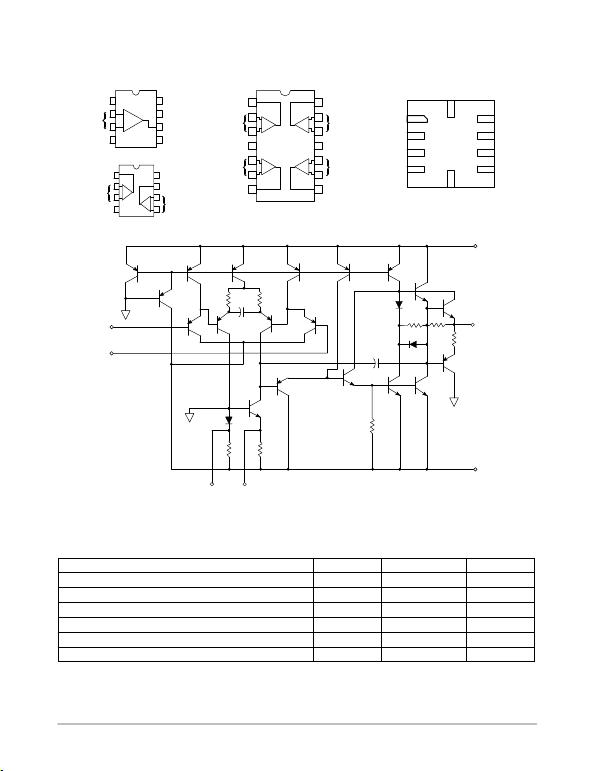MC34071,2,4,A MC33071,2,4,A, NCV33072,4,A
http://onsemi.com
2
CASE 626/CASE 751
PIN CONNECTIONS
(Single, Top View)
(Dual, Top View)
Offset Null
VEE
NC
VCC
Output
Offset Null
Inputs
VEE
Inputs 1
Inputs 2
Output 2
Output 1 VCC
-
1
2
3
4
8
7
6
5
-
-
+
+
1
2
3
4
8
7
6
5
+Inputs 1
Output 1
VCC
Inputs 2
Output 2
Output 4
Inputs 4
VEE
Inputs 3
Output 3
4
23
1
1
2
3
4
5
6
78
9
10
11
12
13
14
-
+
-
+
+
-
+
-
CASE 646/CASE 751A/CASE 948G CASE 510AJ
Output 1
NC
In  1
In + 1 In + 2
In  2
Output 2
NC
VCC
VEE/GND
(Top View)
1
2
3
4
5
6
7
8
9
10
Offset Null
(MC33071, MC34071 only)
Q1
Q2
Q3 Q4 Q5 Q6 Q7
Q17
Q18
D2
C2 D3
R6 R7
R8
R5
Q15 Q16
Q14
Q13
Q11
Q10
R2
C1
R1
Q9
Q8
Q12
D1
R3 R4
Inputs
VCC
Output
Current
Limit
VEE/GND
Base
Current
Cancellation
-
+
Q19
Bias
Figure 1. Representative Schematic Diagram
(Each Amplifier)
MAXIMUM RATINGS
Rating Symbol Value Unit
Supply Voltage (from VEE to VCC) VS+44 V
Input Differential Voltage Range VIDR (Note 1) V
Input Voltage Range VIR (Note 1) V
Output Short Circuit Duration (Note 2) tSC Indefinite Sec
Operating Junction Temperature TJ+150 °C
Storage Temperature Range Tstg 60 to +150 °C
Stresses exceeding Maximum Ratings may damage the device. Maximum Ratings are stress ratings only. Functional operation above the
Recommended Operating Conditions is not implied. Extended exposure to stresses above the Recommended Operating Conditions may affect
device reliability.
1. Either or both input voltages should not exceed the magnitude of VCC or VEE.
2. Power dissipation must be considered to ensure maximum junction temperature (TJ) is not exceeded (see Figure 2).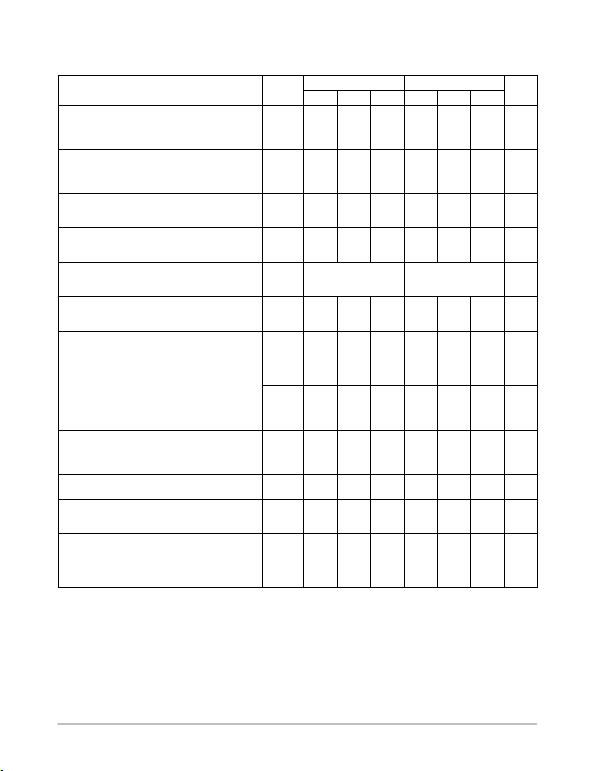MC34071,2,4,A MC33071,2,4,A, NCV33072,4,A
http://onsemi.com
3
ELECTRICAL CHARACTERISTICS (VCC = +15 V, VEE = 15 V, RL = connected to ground, unless otherwise noted. See Note 3 for
TA = Tlow to Thigh)
A Suffix NonSuffix
Characteristics Symbol Min Typ Max Min Typ Max Unit
Input Offset Voltage (RS = 100 W, VCM = 0 V, VO = 0 V)
VCC = +15 V, VEE = 15 V, TA = +25°C
VCC = +5.0 V, VEE = 0 V, TA = +25°C
VCC = +15 V, VEE = 15 V, TA = Tlow to Thigh
VIO
0.5
0.5
3.0
3.0
5.0
1.0
1.5
5.0
5.0
7.0
mV
Average Temperature Coefficient of Input Offset
Voltage
RS = 10 W, VCM = 0 V, VO = 0 V,
TA = Tlow to Thigh
DVIO/DT10 10 mV/°C
Input Bias Current (VCM = 0 V, VO = 0 V)
TA = +25°C
TA = Tlow to Thigh
IIB
100
500
700
100
500
700
nA
Input Offset Current (VCM = 0 V, VO = 0V)
TA = +25°C
TA = Tlow to Thigh
IIO
6.0
50
300
6.0
75
300
nA
Input Common Mode Voltage Range
TA = +25°C
TA = Tlow to Thigh
VICR
VEE to (VCC 1.8)
VEE to (VCC 2.2)
VEE to (VCC 1.8)
VEE to (VCC 2.2)
V
Large Signal Voltage Gain (VO = ±10 V, RL = 2.0 kW)
TA = +25°C
TA = Tlow to Thigh
AVOL
50
25
100
25
20
100
V/mV
Output Voltage Swing (VID = ±1.0 V)
VCC = +5.0 V, VEE = 0 V, RL = 2.0 kW, TA = +25°C
VCC = +15 V, VEE = 15 V, RL = 10 kW, TA = +25°C
VCC = +15 V, VEE = 15 V, RL = 2.0 kW,
TA = Tlow to Thigh
VOH
3.7
13.6
13.4
4.0
14
3.7
13.6
13.4
4.0
14
V
VCC = +5.0 V, VEE = 0 V, RL = 2.0 kW, TA = +25°C
VCC = +15 V, VEE = 15 V, RL = 10 kW, TA = +25°C
VCC = +15 V, VEE = 15 V, RL = 2.0 kW,
TA = Tlow to Thigh
VOL
0.1
14.7
0.3
14.3
13.5
0.1
14.7
0.3
14.3
13.5
V
Output Short Circuit Current (VID = 1.0 V, VO = 0 V,
TA = 25°C)
Source
Sink
ISC
10
20
30
30
10
20
30
30
mA
Common Mode Rejection
RS 10 kW, VCM = VICR, TA = 25°C
CMR 80 97 70 97 dB
Power Supply Rejection (RS = 100 W)
VCC/VEE = +16.5 V/16.5 V to +13.5 V/13.5 V,
TA = 25°C
PSR 80 97 70 97 dB
Power Supply Current (Per Amplifier, No Load)
VCC = +5.0 V, VEE = 0 V, VO = +2.5 V, TA = +25°C
VCC = +15 V, VEE = 15 V, VO = 0 V, TA = +25°C
VCC = +15 V, VEE = 15 V, VO = 0 V,
TA = Tlow to Thigh
ID
1.6
1.9
2.0
2.5
2.8
1.6
1.9
2.0
2.5
2.8
mA
3. Tlow =40°C for MC33071,2,4,/A, NCV33074 Thigh = +85°C for MC33071,2,4,/A, NCV33074
=0°C for MC34071,2,4,/A = +70°C for MC34071,2,4,/A
=40°C for MC34072,4/V, NCV33072,4A = +125°C for MC34072,4/V, NCV33072,4A
Case 510AJ Tlow/Thigh guaranteed by product characterization.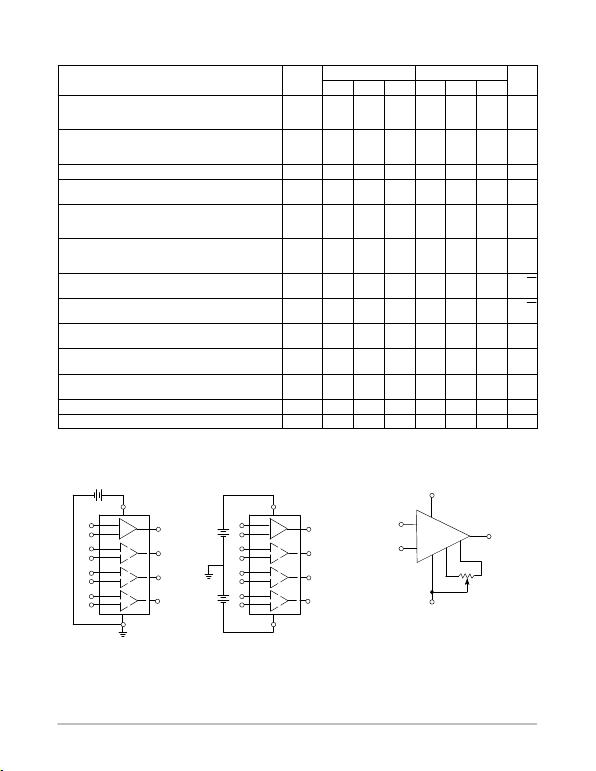MC34071,2,4,A MC33071,2,4,A, NCV33072,4,A
http://onsemi.com
4
AC ELECTRICAL CHARACTERISTICS (VCC = +15 V, VEE = 15 V, RL = connected to ground. TA = +25°C, unless otherwise noted.)
A Suffix NonSuffix
Characteristics Symbol Min Typ Max Min Typ Max Unit
Slew Rate (Vin = 10 V to +10 V, RL = 2.0 kW, CL = 500 pF)
AV = +1.0
AV = 1.0
SR
8.0
10
13
8.0
10
13
V/ms
Setting Time (10 V Step, AV = 1.0)
To 0.1% (+1/2 LSB of 9Bits)
To 0.01% (+1/2 LSB of 12Bits)
ts
1.1
2.2
1.1
2.2
ms
Gain Bandwidth Product (f = 100 kHz) GBW 3.5 4.5 3.5 4.5 MHz
Power Bandwidth
AV = +1.0, RL = 2.0 kW, VO = 20 Vpp, THD = 5.0%
BW 160 160 kHz
Phase margin
RL = 2.0 kW
RL = 2.0 kW, CL = 300 pF
fm
60
40
60
40
Deg
Gain Margin
RL = 2.0 kW
RL = 2.0 kW, CL = 300 pF
Am
12
4.0
12
4.0
dB
Equivalent Input Noise Voltage
RS = 100 W, f = 1.0 kHz
en32 32 nV/ H
z
Equivalent Input Noise Current
f = 1.0 kHz
in0.22 0.22 pA/ H
z
Differential Input Resistance
VCM = 0 V
Rin 150 150 MW
Differential Input Capacitance
VCM = 0 V
Cin 2.5 2.5 pF
Total Harmonic Distortion
AV = +10, RL = 2.0 kW, 2.0 Vpp VO 20 Vpp, f = 10 kHz
THD 0.02 0.02 %
Channel Separation (f = 10 kHz) 120 120 dB
Open Loop Output Impedance (f = 1.0 MHz) |ZO|30 30 W
Figure 2. Power Supply Configurations Figure 3. Offset Null Circuit
Single Supply Split Supplies
1
2
3
4
VCC
VEE
VCC
VCC
VEE
VEE
1
2
3
4
3.0 V to 44 V VCC+|VEE|44 V
Offset nulling range is approximately ±80 mV with a 10 k
potentiometer (MC33071, MC34071 only).
VCC
VEE
1
2
3
4
5
6
7
10 k
+
-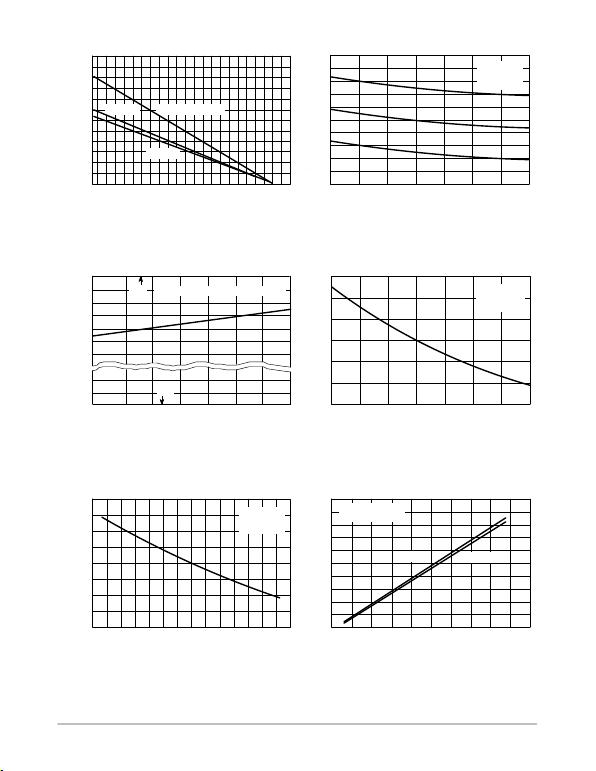MC34071,2,4,A MC33071,2,4,A, NCV33072,4,A
http://onsemi.com
5
RL Connected
to Ground TA = 25°C
RL = 10 k RL = 2.0 k
VO, OUTPUT VOLTAGE SWING (Vpp)
Figure 4. Maximum Power Dissipation versus
Temperature for Package Types
Figure 5. Input Offset Voltage versus
Temperature for Representative Units
Figure 6. Input Common Mode Voltage
Range versus Temperature
Figure 7. Normalized Input Bias Current
versus Temperature
Figure 8. Normalized Input Bias Current versus
Input Common Mode Voltage
Figure 9. Split Supply Output Voltage
Swing versus Supply Voltage
TA, AMBIENT TEMPERATURE (°C)
D
P, MAXIMUM POWER DISSIPATION (mW)
-55 -40 -20 0 20 40 60 80 100 120 140 160
8 & 14 Pin Plastic Pkg
SOIC-14 Pkg
SOIC-8 Pkg
TA, AMBIENT TEMPERATURE (°C)
IO
V, INPUT OFFSET VOLTAGE (mV)
-55 -25 0 25 50 75 100 125
VCC = +15 V
VEE = -15 V
VCM = 0
TA, AMBIENT TEMPERATURE (°C)
ICR
V, INPUT COMMON MODE VOLTAGE RANGE (V)
-55 -25 0 25 50 75 100 125
VCC VCC/VEE = +1.5 V/ -1.5 V to +22 V/ -22 V
VEE
TA, AMBIENT TEMPERATURE (°C)
IB
I, INPUT BIAS CURRENT (NORMALIZED)
-55 -25 0 25 50 75 100 125
VCC = +15 V
VEE = -15 V
VCM = 0
VIC, INPUT COMMON MODE VOLTAGE (V)
-12 -8.0 -4.0 0 4.0 8.0 12
VCC = +15 V
VEE = -15 V
TA = 25°C
VCC, |VEE|, SUPPLY VOLTAGE (V)
0 5.0 10 15 20 25
V
IB
I, INPUT BIAS CURRENT (NORMALIZED)
2400
2000
1600
1200
800
400
0
4.0
2.0
0
-2.0
-4.0
VCC
VCC -0.8
VCC -1.6
VCC -2.4
VEE +0.01
VEE
1.3
1.2
1.1
1.0
0.9
0.8
0.7
1.4
1.2
1.0
0.8
0.6
50
40
30
20
10
0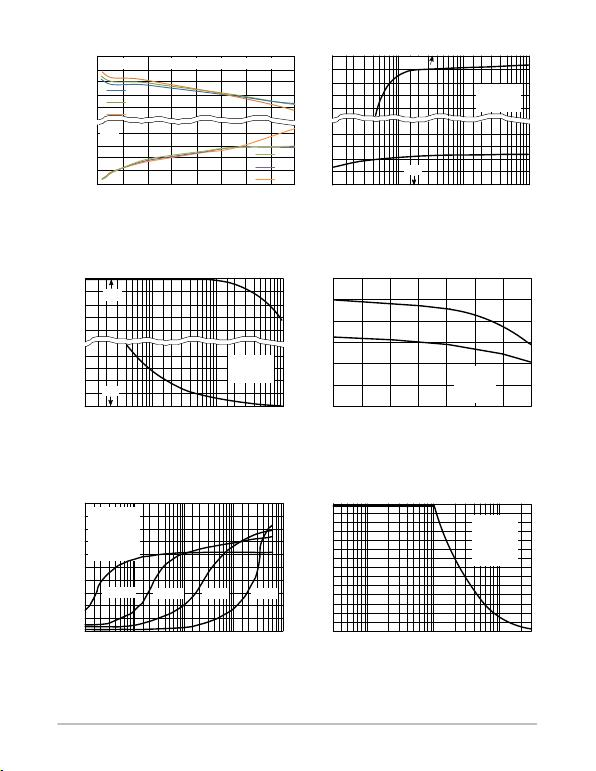MC34071,2,4,A MC33071,2,4,A, NCV33072,4,A
http://onsemi.com
6
VCC
VCC = +15 V
RL to VCC
TA = 25°C
GND
VCC
VCC = +15 V
RL = GND
TA = 25°C
GND
VO, OUTPUT VOLTAGE SWING (Vpp)
Figure 10. Split Supply Output Saturation
60
Figure 11. Single Supply Output Saturation
Figure 12. Single Supply Output Saturation
Figure 13. Output Short Circuit Current
versus Temperature
Figure 14. Output Impedance
versus Frequency
Figure 15. Output Voltage Swing
versus Frequency
0 5.0 10 15 20
Sink
VCC/VEE = +4.5 V/ -4.5 V to +22 V/ -22 V
Source
RL, LOAD RESISTANCE TO GROUND (W)
100 1.0 k 10 k 100 k
sat
V , OUTPUT SATURATION VOLTAGE (V)
RL, LOAD RESISTANCE TO VCC (W)
100 1.0 k 10 k 100 k
TA, AMBIENT TEMPERATURE (°C)
SC
I, OUTPUT CURRENT (mA)
-55 -25 0 25 50 75 100 125
VCC = +15 V
VEE = -15 V
RL 0.1 W
DVin = 1.0 V
Sink
Source
f, FREQUENCY (Hz)
O
Z, OUTPUT IMPEDANCE ()Ω
1.0 k 10 k 100 1.0 M 10 M
AV = 1000 AV = 100 AV = 10 AV = 1.0
VCC = +15 V
VEE = -15 V
VCM = 0
VO = 0
DIO = ±0.5 mA
TA = 25°C
f, FREQUENCY (Hz)
3.0 k 10 k 30 k 100 k 300 k 1.0 M 3.0 M
VCC = +15 V
VEE = -15 V
AV = +1.0
RL = 2.0 k
THD 1.0%
TA = 25°C
sat
V , OUTPUT SATURATION VOLTAGE (V)
sat
V , OUTPUT SATURATION VOLTAGE (V)
VCC
VCC -1.0
VCC -2.0
VEE +2.0
VEE +1.0
VEE
VCC-2.0
VCC-4.0
VCC
0.2
0.1
0
0
-0.4
-0.8
2.0
1.0
50
40
30
20
10
0
50
40
30
20
10
0
28
24
20
16
12
8.0
4.0
0
40
25
25
85
125
125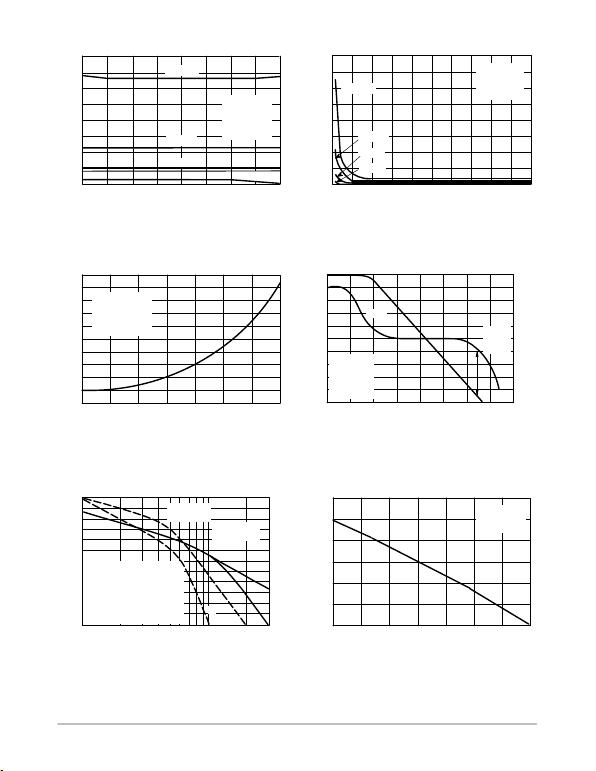MC34071,2,4,A MC33071,2,4,A, NCV33072,4,A
http://onsemi.com
7
1. Phase RL = 2.0 k
2. Phase RL = 2.0 k, CL = 300 pF
3. Gain RL = 2.0 k
4. Gain RL = 2.0 k, CL = 300 pF
VCC = +15 V
VEE = 15 V
VO = 0 VTA = 25°C
Phase
Margin = 60°
Gain
Margin = 12 dB
3
4
1
2
Gain
VCC = +15 V
VEE = -15 V
VO = 0 V
RL = 2.0 k
TA = 25°C
Phase
Phase
Margin
= 60°
Figure 16. Total Harmonic Distortion
versus Frequency
Figure 17. Total Harmonic Distortion
versus Output Voltage Swing
Figure 18. Open Loop Voltage Gain
versus Temperature
Figure 19. Open Loop Voltage Gain and
Phase versus Frequency
Figure 20. Open Loop Voltage Gain and
Phase versus Frequency
Figure 21. Normalized Gain Bandwidth
Product versus Temperature
f, FREQUENCY (Hz)
10 100 1.0 k 10 k 100 k
AV = 1000
AV = 100
AV = 10
AV = 1.0
VCC = +15 V
VEE = -15 V
VO = 2.0 Vpp
RL = 2.0 k
TA = 25°C
VO, OUTPUT VOLTAGE SWING (Vpp)
THD, TOTAL HARMONIC DISTORTION (%)
0 4.0 8.0 12 16 20
VCC = +15 V
VEE = -15 V
RL = 2.0 k
TA = 25°C
AV = 1000
AV = 100
AV = 10
AV = 1.0
TA, AMBIENT TEMPERATURE (°C)
-55 -25 0 25 50 75 100 125
VCC = +15 V
VEE = -15 V
VO= -10 V to +10 V
RL = 10 k
f 10Hz
f, FREQUENCY (Hz)
1.0 10 100 1.0 k 10 k 100 k 1.0 M 10 M 100 M
, EXCESS PHASE (DEGREES)
φ
, EXCESS PHASE (DEGREES)
φ
f, FREQUENCY (MHz)
1.0 2.0 3.0 5.0 7.0 10 20 30
TA, AMBIENT TEMPERATURE (°C)
GBW, GAIN BANDWIDTH PRODUCT (NORMALIED)
-55 -25 0 25 50 75 100 125
VCC = +15 V
VEE = -15 V
RL = 2.0 k
VOL
A, OPEN LOOP VOLTAGE GAIN (dB)
0.4
0.3
0.2
0.1
0
4.0
3.0
2.0
1.0
0
116
112
108
104
100
96
100
80
60
40
20
0
20
10
0
-10
-20
-30
-40
1.15
1.1
1.05
1.0
0.95
0.9
0.85
0
45
90
135
180
100
120
140
160
180
THD, TOTAL HARMONIC DISTORTION (%)
VOL
A, OPEN LOOP VOLTAGE GAIN (dB)
VOL
A, OPEN LOOP VOLTAGE GAIN (dB)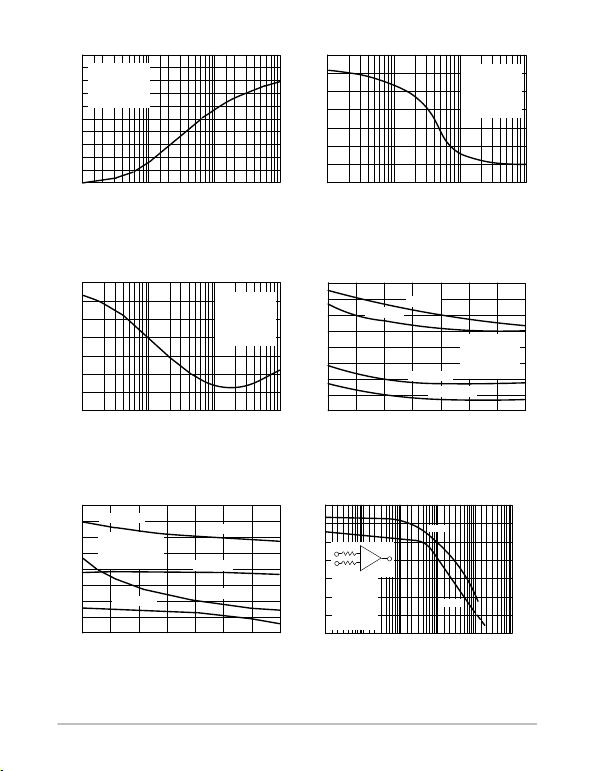MC34071,2,4,A MC33071,2,4,A, NCV33072,4,A
http://onsemi.com
8
VCC = +15 V
VEE = -15 V
AV = +1.0
RL = 2.0 k to R
VO = -10 V to +10 V
TA = 25°C
Figure 22. Percent Overshoot versus
Figure 23. Phase Margin versus
Figure 24. Gain Margin versus Load Capacitance Figure 25. Phase Margin versus Temperature
Figure 26. Gain Margin versus Temperature Figure 27. Phase Margin and Gain Margin
versus Differential Source Resistance
PERCENT OVERSHOOT
10 100 1.0 k 10 k
VCC = +15 V
VEE = -15 V
RL = 2.0 k
VO = -10 V to +10 V
TA = 25°C
, PHASE MARGIN (DEGREES)φm
10 100 1.0 k 10 k
VCC = +15 V
VEE = -15 V
AV = +1.0
RL = 2.0 k to
VO = -10 V to +10 V
TA = 25°C
m
A, GAIN MARGIN (dB)
10 100 1.0 k 10 k
, PHASE MARGIN (DEGREES)φm
TA, AMBIENT TEMPERATURE (°C)
-55 -25 0 25 50 75 100 125
VCC = +15 V
VEE = -15 V
AV = +1.0
RL = 2.0 k to
VO = -10 V to +10 V
CL = 10 pF
CL = 100 pF
CL = 1,000 pF
CL = 10,000 pF
TA, AMBIENT TEMPERATURE (°C)
-55 -25 0 25 50 75 100 125
VCC = +15 V
VEE = -15 V
AV = +1.0
RL = 2.0 k to
VO = -10 V to +10 V
CL = 10 pF
CL = 1,000 pF
m
A, GAIN MARGIN (dB)
CL = 100 pF
CL = 10,000 pF
Phase
m
A, GAIN MARGIN (dB)
RT, DIFFERENTIAL SOURCE RESISTANCE (W)
1.0 100 1.0 k 10 k10 100 k
R1
R2
VO
+
-
VCC = +15 V
VEE = -15 V
RT = R1 + R2
AV = +100
VO = 0 V
TA = 25°C
Gain
, PHASE MARGIN (DEGREES)φm
100
80
60
40
20
0
70
60
50
40
30
20
10
0
14
12
10
8.0
6.0
2.0
0
4.0
80
60
40
20
0
16
12
8.0
4.0
0
12
10
8.0
6.0
4.0
2.0
0
60
50
40
30
20
10
0
70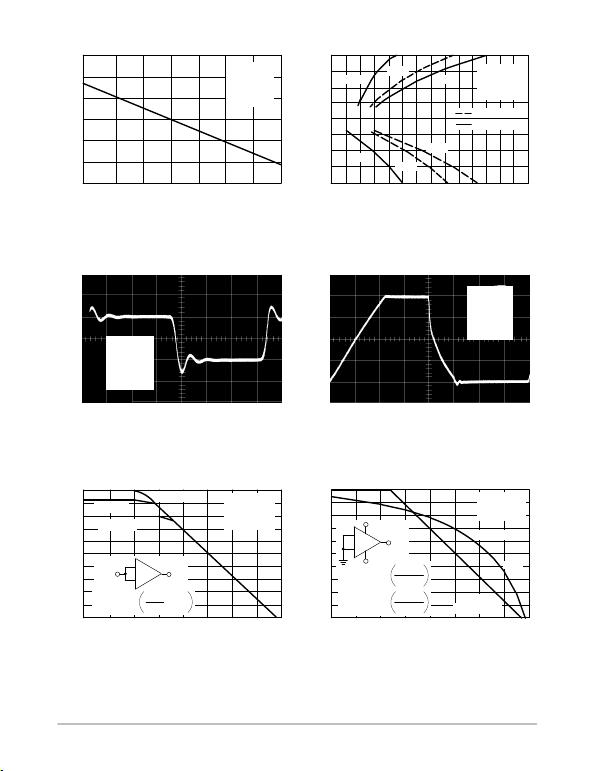MC34071,2,4,A MC33071,2,4,A, NCV33072,4,A
http://onsemi.com
9
Figure 28. Normalized Slew Rate
versus Temperature
Figure 29. Output Settling Time
Figure 30. Small Signal Transient Response Figure 31. Large Signal Transient Response
Figure 32. Common Mode Rejection
versus Frequency
Figure 33. Power Supply Rejection
versus Frequency
TA, AMBIENT TEMPERATURE (°C)
SR, SLEW RATE (NORMALIZED)
-55 -25 0 25 50 75 100 125
VCC = +15 V
VEE = -15 V
AV = +1.0
RL = 2.0 k
CL = 500 pF
ts, SETTLING TIME (ms)
O
V, OUTPUT VOLTAGE SWING FROM 0 V (V)
Δ
0 0.5 1.0 1.5 2.0 2.5 3.0 3.5
VCC = +15 V
VEE = -15 V
AV = -1.0
TA = 25°C
10 mV
1.0 mV
1.0 mV
Compensated
Uncompensated
10 mV
1.0 mV
1.0 mV
50 mV/DIV
2.0 ms/DIV
VCC = +15 V
VEE = -15 V
AV = +1.0
RL = 2.0 k
CL = 300 pF
TA = 25°C
5.0 V/DIV
1.0 ms/DIV
f, FREQUENCY (Hz)
CMR, COMMON MODE REJECTION (dB)
0.1 1.0 10 100 1.0 k 10 k 100 k 1.0 M 10 M
TA = 25°C
TA = 125°C
TA = -55°C
VCC = +15 V
VEE = -15 V
VCM = 0 V
DVCM = ±1.5 V
f, FREQUENCY (Hz)
PSR, POWER SUPPLY REJECTION (dB)
0.1 1.0 10 100 1.0 k 10 k 100 k 1.0 M 10 M
VCC = +15 V
VEE = -15 V
TA = 25°C
(DVCC = +1.5 V)
(DVEE = +1.5 V)
+PSR
-PSR
VCC = +15 V
VEE = -15 V
AV = +1.0
RL = 2.0 k
CL = 300 pF
TA = 25°C
1.15
1.1
1.05
1.0
0.95
0.9
0.85
10
5.0
0
-5.0
-10
00
100
80
60
40
20
0
100
80
60
40
20
0
DVCM DVO
CMR = 20 Log DVCM
DVO
+
-
DVO
+
-
DVCC
DVEE
DVCC
+PSR = 20 Log
DVEE
-PSR = 20 Log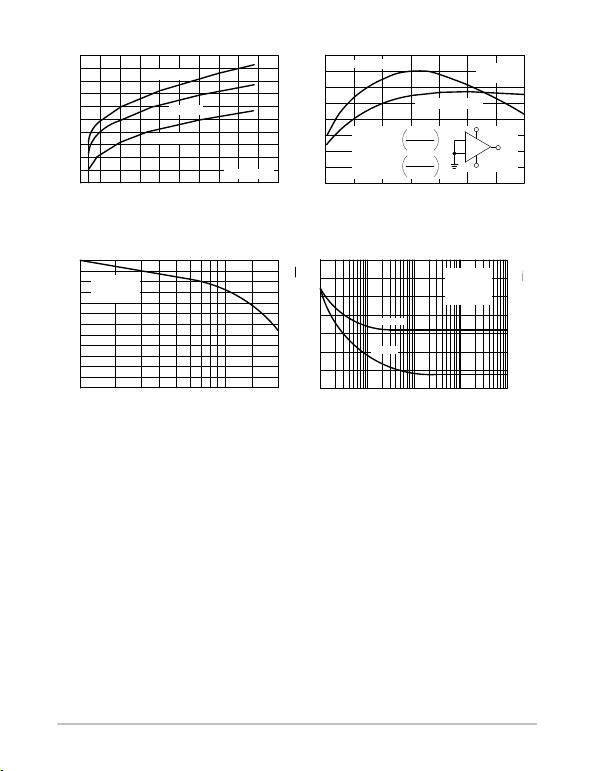MC34071,2,4,A MC33071,2,4,A, NCV33072,4,A
http://onsemi.com
10
Figure 34. Supply Current versus
Supply Voltage
Figure 35. Power Supply Rejection
versus Temperature
Figure 36. Channel Separation versus Frequency Figure 37. Input Noise versus Frequency
VCC, |VEE|, SUPPLY VOLTAGE (V)
CC
I , SUPPLY CURRENT (mA)
0 5.0 10 15 20 25
TA = 25°C
TA = 125°C
TA = -55°C
TA, AMBIENT TEMPERATURE (°C)
PSR, POWER SUPPLY REJECTION (dB)
-55 -25 0 25 50 75 100 125
VCC = +15 V
VEE = -15 V
(DVCC = +1.5 V)
(DVEE = +1.5 V)
+PSR
-PSR
f, FREQUENCY (kHz)
CHANNEL SEPARATION (dB)
10 20 30 50 70 100 200 300
VCC = +15 V
VEE = -15 V
TA = 25°C
f, FREQUENCY (kHz)
n
e, INPUT NOICE VOLTAGE (
i, INPUT NOISE CURRENT (pA )
10 100 1.0 k 10 k 100 k
nV Hz)
Hz
n
Voltage
Current
9.0
8.0
7.0
6.0
5.0
4.0
105
95
85
75
65
120
100
80
60
40
20
0
70
60
50
40
30
20
10
0
2.8
2.4
2.0
1.6
1.2
0.8
0.4
0
DVO
+
-
DVCC
DVEE
DVCC
+PSR = 20 Log
DVEE
-PSR = 20 Log
VCC = +15 V
VEE = -15 V
VCM = 0
TA = 25°C
APPLICATIONS INFORMATION
CIRCUIT DESCRIPTION/PERFORMANCE FEATURES
Although the bandwidth, slew rate, and settling time of the
MC34071 amplifier series are similar to op amp products
utilizing JFET input devices, these amplifiers offer other
transistor differential input stage and an all NPN transistor
output stage.
Since the input common mode voltage range of this input
stage includes the VEE potential, single supply operation is
feasible to as low as 3.0 V with the common mode input
voltage at ground potential.
The input stage also allows differential input voltages up
to ±44 V, provided the maximum input voltage range is not
exceeded. Specifically, the input voltages must range
between VEE and VCC supply voltages as shown by the
maximum rating table. In practice, although not
recommended, the input voltages can exceed the VCC
voltage by approximately 3.0 V and decrease below the VEE
voltage by 0.3 V without causing product damage, although
output phase reversal may occur. It is also possible to source
up to approximately 5.0 mA of current from VEE through
either inputs clamping diode without damage or latching,
although phase reversal may again occur.
If one or both inputs exceed the upper common mode
voltage limit, the amplifier output is readily predictable and
may be in a low or high state depending on the existing input
bias conditions.
Since the input capacitance associated with the small
geometry input device is substantially lower (2.5 pF) than
the typical JFET input gate capacitance (5.0 pF), better
frequency response for a given input source resistance can
be achieved using the MC34071 series of amplifiers. This
performance feature becomes evident, for example, in fast
settling DtoA current to voltage conversion applications
where the feedback resistance can form an input pole with
the input capacitance of the op amp. This input pole creates
a 2nd order system with the single pole op amp and is
therefore detrimental to its settling time. In this context,
lower input capacitance is desirable especially for higherMC34071,2,4,A MC33071,2,4,A, NCV33072,4,A
http://onsemi.com
11
values of feedback resistances (lower current DACs). This
input pole can be compensated for by creating a feedback
zero with a capacitance across the feedback resistance, if
necessary, to reduce overshoot. For 2.0 kW of feedback
resistance, the MC34071 series can settle to within 1/2 LSB
of 8bits in 1.0 ms, and within 1/2 LSB of 12bits in 2.2 ms
for a 10 V step. In a inverting unity gain fast settling
configuration, the symmetrical slew rate is ±13 V/ms. In the
classic noninverting unity gain configuration, the output
positive slew rate is +10 V/ms, and the corresponding
negative slew rate will exceed the positive slew rate as a
function of the fall time of the input waveform.
Since the bipolar input device matching characteristics
are superior to that of JFETs, a low untrimmed maximum
offset voltage of 3.0 mV prime and 5.0 mV downgrade can
be economically offered with high frequency performance
characteristics. This combination is ideal for low cost
precision, high speed quad op amp applications.
The all NPN output stage, shown in its basic form on the
equivalent circuit schematic, offers unique advantages over
the more conventional NPN/PNP transistor Class AB output
stage. A 10 kW load resistance can swing within 1.0 V of the
positive rail (VCC), and within 0.3 V of the negative rail
(VEE), providing a 28.7 Vpp swing from ±15 V supplies.
This large output swing becomes most noticeable at lower
supply voltages.
The positive swing is limited by the saturation voltage of
the current source transistor Q7, and VBE of the NPN pull up
transistor Q17, and the voltage drop associated with the short
circuit resistance, R7. The negative swing is limited by the
saturation voltage of the pulldown transistor Q16, the
voltage drop ILR6, and the voltage drop associated with
resistance R7, where IL is the sink load current. For small
valued sink currents, the above voltage drops are negligible,
allowing the negative swing voltage to approach within
millivolts of VEE. For large valued sink currents (>5.0 mA),
diode D3 clamps the voltage across R6, thus limiting the
negative swing to the saturation voltage of Q16, plus the
forward diode drop of D3 (VEE +1.0 V). Thus for a given
supply voltage, unprecedented peaktopeak output voltage
swing is possible as indicated by the output swing
specifications.
ground for single supply applications, the maximum
possible output swing can be achieved for a given supply
the output to VCC during the positive swing and the output
will pull the load resistance near ground during the negative
swing. The load resistance value should be much less than
that of the feedback resistance to maximize pull up
capability.
Because the PNP output emitterfollower transistor has
been eliminated, the MC34071 series offers a 20 mA
minimum current sink capability, typically to an output
voltage of (VEE +1.8 V). In single supply applications the
output can directly source or sink base current from a
common emitter NPN transistor for fast high current
switching applications.
In addition, the all NPN transistor output stage is
inherently fast, contributing to the bipolar amplifiers high
gain bandwidth product and fast settling capability. The
associated high frequency low output impedance (30 W typ
@ 1.0 MHz) allows capacitive drive capability from 0 pF to
10,000 pF without oscillation in the unity closed loop gain
configuration. The 60° phase margin and 12 dB gain margin
as well as the general gain and phase characteristics are
virtually independent of the source/sink output swing
conditions. This allows easier system phase compensation,
since output swing will not be a phase consideration. The
high frequency characteristics of the MC34071 series also
allow excellent high frequency active filter capability,
especially for low voltage single supply applications.
Although the single supply specifications is defined at
5.0 V, these amplifiers are functional to 3.0 V @ 25°C
although slight changes in parametrics such as bandwidth,
slew rate, and DC gain may occur.
If power to this integrated circuit is applied in reverse
polarity or if the IC is installed backwards in a socket, large
unlimited current surges will occur through the device that
may result in device destruction.
Special static precautions are not necessary for these
bipolar amplifiers since there are no MOS transistors on the
die.
As with most high frequency amplifiers, proper lead
dress, component placement, and PC board layout should be
exercised for optimum frequency performance. For
example, long unshielded input or output leads may result in
unwanted inputoutput coupling. In order to preserve the
relatively low input capacitance associated with these
amplifiers, resistors connected to the inputs should be
stray input capacitance. This not only minimizes the input
pole for optimum frequency response, but also minimizes
extraneous “pick up” at this node. Supply decoupling with
is also important, particularly over temperature, since many
types of decoupling capacitors exhibit great impedance
changes over temperature.
The output of any one amplifier is current limited and thus
protected from a direct short to ground. However, under
such conditions, it is important not to allow the device to
exceed the maximum junction temperature rating. Typically
for ±15 V supplies, any one output can be shorted
continuously to ground without exceeding the maximum
temperature rating.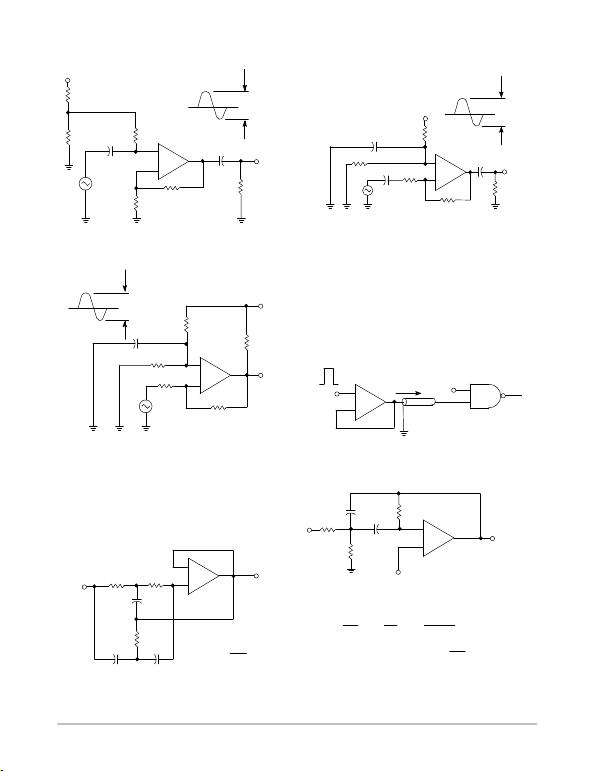MC34071,2,4,A MC33071,2,4,A, NCV33072,4,A
http://onsemi.com
12
Figure 38. AC Coupled Noninverting Amplifier Figure 39. AC Coupled Inverting Amplifier
(Typical Single Supply Applications VCC = 5.0 V)
Figure 40. DC Coupled Inverting Amplifier
Maximum Output Swing
Figure 41. Unity Gain Buffer TTL Driver
Figure 42. Active HighQ Notch Filter Figure 43. Active Bandpass Filter
-
+
VCC
5.1 M
20 k Cin
Vin
1.0 M
MC34071
VO
03.7 Vpp
RL
10 k
AV = 101
100 k
1.0 k
BW (-3.0 dB) = 45 kHz
COVO
36.6 mVpp
-
+
3.7 Vpp
0
VCC
VO
100 k
Cin 10 k
100 k
CO
RL
10 k
68 k
Vin 370 mVpp
AV = 10 BW (-3.0 dB) = 450 kHz
+
-
4.75 Vpp
VO
VO
VCC
RL
100 k
91 k
5.1 k
1.0 M
AV = 10
Vin
2.63 V
5.1 k
BW (-3.0 dB) = 450 kHz
-
+
Vin
2.5 V
0 0 to 10,000 pF
Cable TTL Gate
-
+
Vin
VO
16 k
C
0.01
32 k 2.0 R
2.0 C
0.02
fo = 1.0 kHz
fo =
Vin 0.2 Vdc
1
4pRC
2.0 C
0.02
16 k
RR
-
+
Vin VO
VCC
R3
2.2 k
C
0.047
R2
5.6 k
0.4 VCC
R1
fo = 30 kHz
Ho = 10
Ho = 1.0
1.1 k
Given fo = Center Frequency
AO = Gain at Center Frequency
Choose Value fo, Q, Ao, C
R3 =  R1 =  R2 =
Q R3 R1 R3
2Ho4Q2R1-R3
pfoC
For less than 10% error from operational amplifier
Qofo
GBW < 0.1
where fo and GBW are expressed in Hz.
C
0.047
MC34071
MC34071
MC34071
MC34071
MC34071
MC54/74XX
Then:
GBW = 4.5 MHz Typ.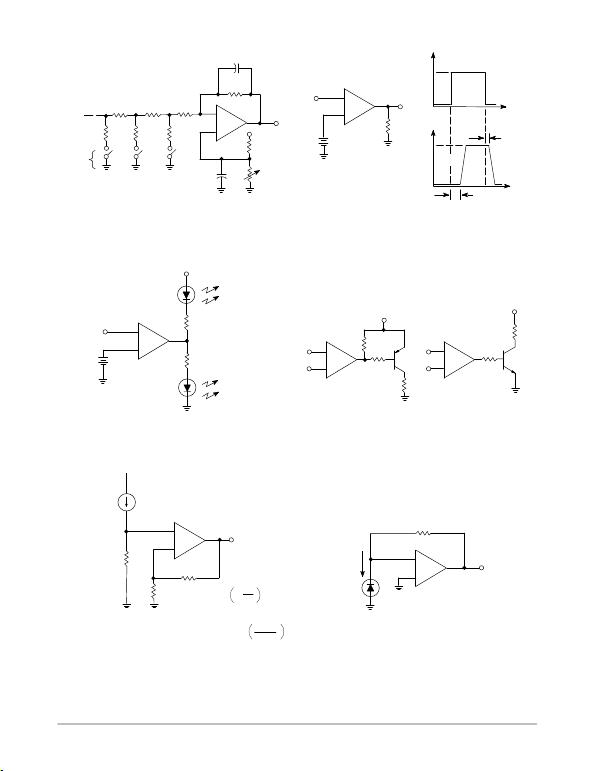MC34071,2,4,A MC33071,2,4,A, NCV33072,4,A
http://onsemi.com
13
Figure 44. Low Voltage Fast D/A Converter Figure 45. High Speed Low Voltage Comparator
Figure 46. LED Driver Figure 47. Transistor Driver
Figure 48. AC/DC Ground Current Monitor Figure 49. Photovoltaic Cell Amplifier
5.0 k
10 k
Bit
Switches
CF
RF
VO
VCC
Settling Time
1.0 ms (8-Bits, 1/2 LSB)
-
+
5.0 k5.0 k
10 k 10 k
-
+
VO
VO
Vin
1.0 V
2.0 k
RL
2.0 V
4.0 V
0.1
t
25 V/ms
0.2 ms
Delay
Delay
1.0 ms
Vin
t
13 V/ms
-
+
VCC
Vref
“ON"
Vin < Vref
“ON"
Vin > Vref
Vin
-
+
VCC
VCC
RL
RL
(A) PNP (B) NPN
-
+
-
+
VO
R1
R2
RS
Ground Current
Sense Resistor
BW ( -3.0 dB) = GBW
For VO > 0.1V
R1
R2
R1+R2
R2
-
+
VO
MC34071
ICell
VCell = 0 V
VO = ICell RF
VO > 0.1 V
RF
1+
MC34071
MC34071
MC34071 MC34071
MC34071
MC34071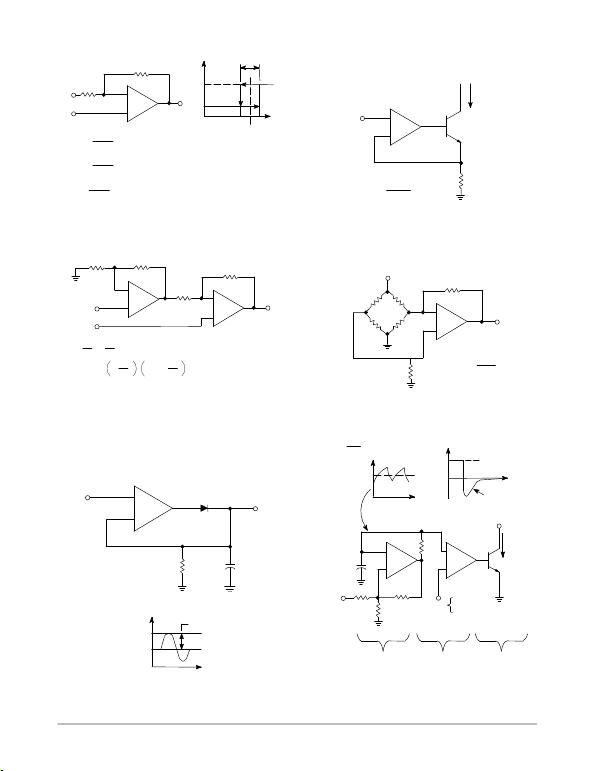MC34071,2,4,A MC33071,2,4,A, NCV33072,4,A
http://onsemi.com
14
Figure 50. Low Input Voltage Comparator
with Hysteresis
Figure 51. High Compliance Voltage to
Sink Current Converter
Figure 52. High Input Impedance
Differential Amplifier
Figure 53. Bridge Current Amplifier
Figure 54. Low Voltage Peak Detector Figure 55. High Frequency Pulse
Width Modulation
Vref
R2
VO
VOH
VOL
VinL VinH
Vref
Hysteresis
Vin
Vin
R1
MC34071
VinL =(V
OL-Vref)+Vref
R1
R1+R2
VinH =(V
OH-Vref)+Vref
VH =(V
OH -VOL)
+
-
R1
R1+R
R1
R1+R2
Vin
Iout
R
-
+
Iout = Vin±VIO
R
1/2
MC34072 -
+
+
R1 R2
R3
R4
VO
+V1
+V2
R2 R4
R3R1 (Critical to CMRR)
VO = 1 V2-V1
For (V2 V1), V > 0
=
-
+R4
R3
R4
R3
-
+
+Vref
RF
VO
RR
R
R = DR
DR < < R
RF > > R (VO 0.1 V)
RF
VO = Vref
DR RF
2R2
-
+
Vin
Vin
RLVP10,000 pF
VO = Vin (pk)
+
VP
t
-+
+
VP
t
t
Iout
VP
+
-
0
+
ISC
Base Charge
Removal
±IB
V+
47 k
100 k
C
R
Pulse Width
Control Group
OSC Comparator High Current
Output
fOSC ^
V
0.85
RC
-
100 k
IB
MC34071
MC34071
MC34071
1/2
MC34072
1/2
MC34072
1/2
MC34072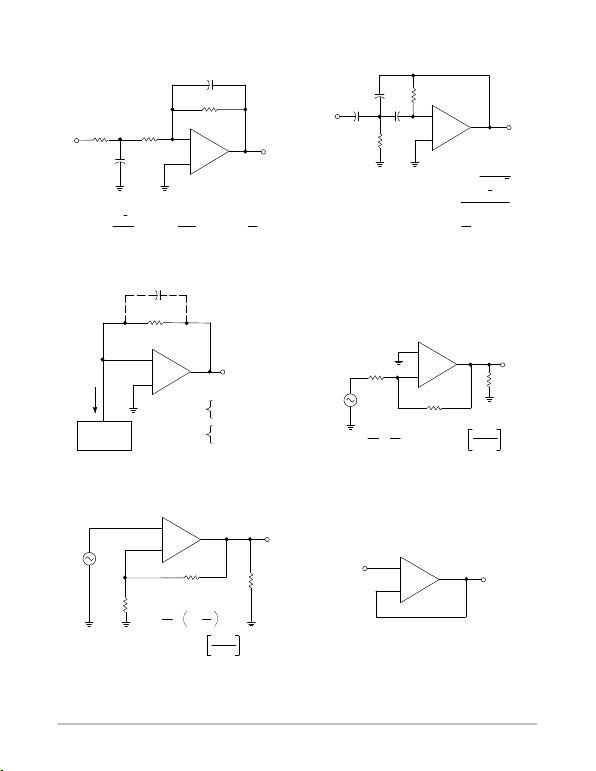MC34071,2,4,A MC33071,2,4,A, NCV33072,4,A
http://onsemi.com
15
Figure 56. Second Order LowPass Active Filter Figure 57. Second Order HighPass Active Filter
GENERAL ADDITIONAL APPLICATIONS INFORMATION VS = ±15.0 V
Figure 58. Fast Settling Inverter Figure 59. Basic Inverting Amplifier
Figure 60. Basic Noninverting Amplifier Figure 61. Unity Gain Buffer (AV = +1.0)
-
+
R1 R3
560 510
C2
C1
0.44
0.02
R2
5.6 k
MC34071
fo = 1.0 kHz
Ho = 10
Choose: fo, Ho, C2
Then: C1 = 2C2 (Ho+1)
R2 = R3 = R1 =
R2
Ho
Ho+1
4pfoC2
R2
+
-
C2
0.05 C1
1.0
R1
46.1 k
R2
1.1 k
fo = 100 Hz
Ho = 20
Choose: fo, Ho, C1 Then: R1 =
R2 =
C2 =
Ho+0.5
pfoC1
2pfoC1 (1/Ho+2)
C
Ho
C1
1.0
+
-
CF*
VO = 10 V
Step
RF
2.0 k
I
High Speed
DAC
*Optional Compensation
Uncompensated
Compensated
ts = 1.0 ms
to 1/2 LSB (8-Bits)
ts = 2.2 ms
to 1/2 LSB (12-Bits)
SR = 13 V/ms
VO
+
-
R1
R2
VO
Vin
RL
BW (-3.0 dB) = GBW
=
SR = 13 V/ms
VO
Vin
R2
R1 R1 +R2
R1
BW (-3.0 dB) = GBW R1 +R2
R1
+
-
Vin
VO
R2
RL
R1
=
VO
Vin
R2
R1
1 +
+
-
Vin
VO
BWp = 200 kHz
VO = 20 Vpp
SR = 10 V/ms
MC34071
MC34071
MC34071
MC34071
MC34071
2
Ǹ
2
Ǹ
2
Ǹ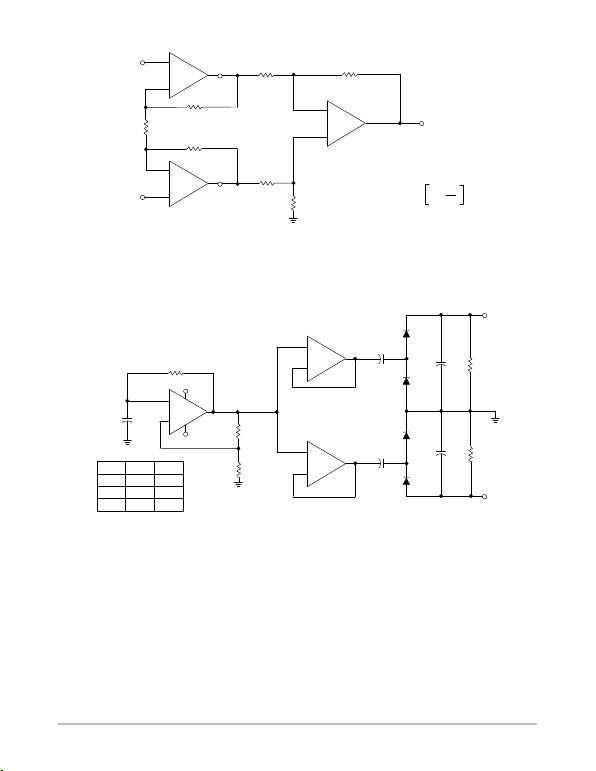MC34071,2,4,A MC33071,2,4,A, NCV33072,4,A
http://onsemi.com
16
Figure 62. High Impedance Differential Amplifier
Figure 63. Dual Voltage Doubler
-
R
RE
Example:
Let: R = RE = 12 k
Then: AV = 3.0
BW = 1.5 MHz
AV = 1 +2 R
RE
-
-
+
+
+
VO
R
R
RR
R
MC34074
-
+
+
100 k 10
+10
-10
220 pF
-VO
+VO
RL+VO-VO
18.93 -18.78
10 k 18 -18
5.0 k 15.4 -15.4
RL
100 k
100 k
RL
+
+
-
+
+
+
10
10
10
-
MC34074
MC34074
MC34074
MC34074
MC34074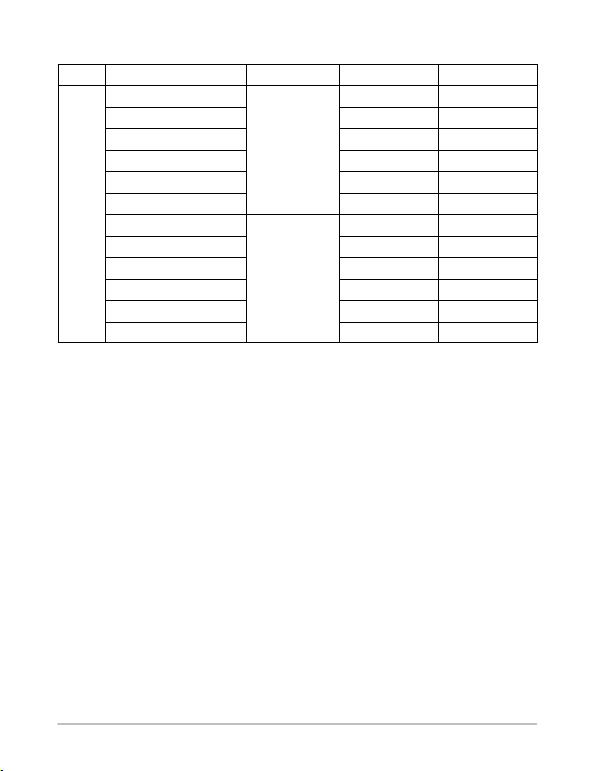MC34071,2,4,A MC33071,2,4,A, NCV33072,4,A
http://onsemi.com
17
ORDERING INFORMATION
Op Amp
Function Device
Operating
Temperature Range Package Shipping
Single
MC34071PG
TA = 0° to +70°C
PDIP8
(PbFree) 50 Units / Rail
MC34071APG PDIP8
(PbFree) 50 Units / Rail
MC34071DG SOIC8
(PbFree) 98 Units / Rail
MC34071DR2G SOIC8
(PbFree) 2500 / Tape & Reel
(PbFree) 98 Units / Rail
(PbFree) 2500 / Tape & Reel
MC33071PG
TA = 40° to +85°C
PDIP8
(PbFree) 50 Units / Rail
MC33071APG PDIP8
(PbFree) 50 Units / Rail
MC33071DG SOIC8
(PbFree) 98 Units / Rail
MC33071DR2G SOIC8
(PbFree) 2500 / Tape & Reel